# Neural networks from scratch in Scala!¶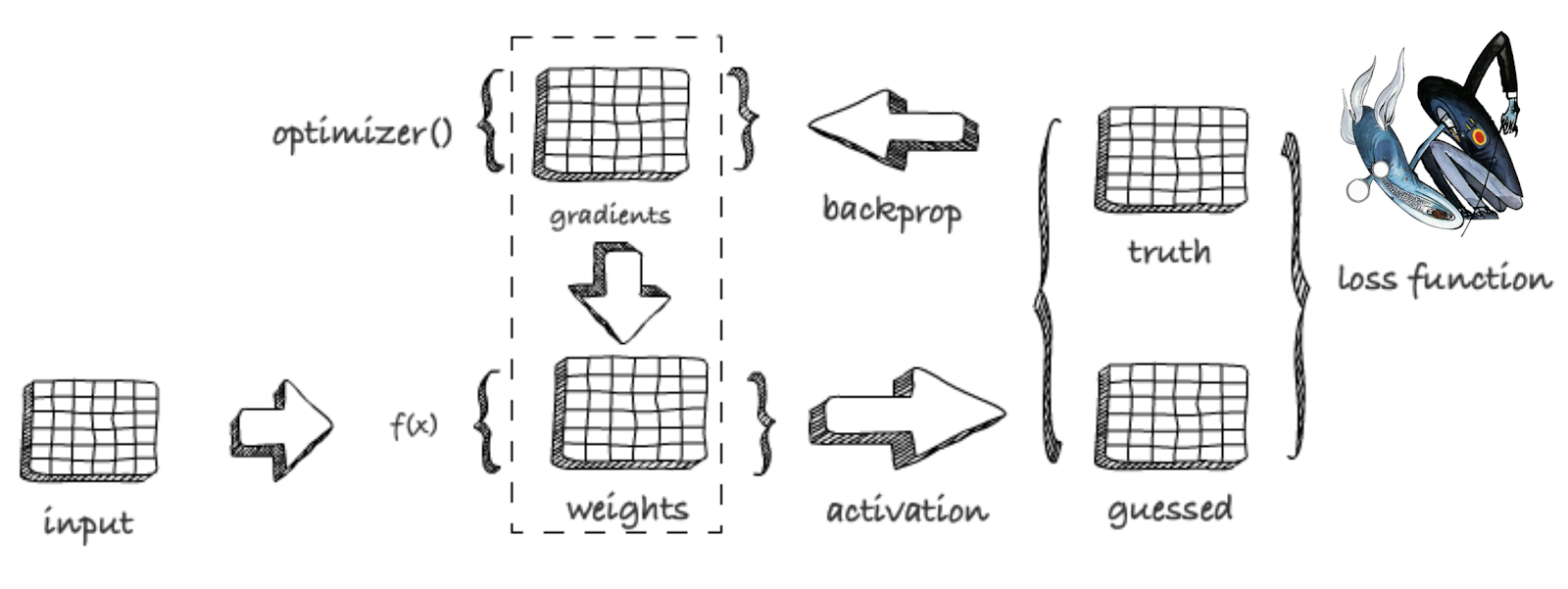This PolyNotebook explains how to implement a simple neural network in Scala.

PolyNote is the polyglot notebook from Netflix. Check it out at https://polynote.org/.

First some jargon.

In its simplest form, a neural net is a sequence of linear transformations (read matrix multiplications) glued together by activation functions. The task of the activation function is to introduce a non-linearity (such as setting the negative values to 0) between these transformations. The reason this works can be traced back to the Universal Approximation Theorem. The matrices are called weights, or parameters. The linear transformation together with the activation function is called a layer. Layers can be stacked together to form modules. The complete set of modules that compose the neural net is often called a model.

The neural net we'll be discussing is part of the supervised domain of machine learning. In supervised learning there is a learning, or training phase, and an inference phase.

In the training phase, a dataloader pushes labeled input in the form of a matrix (input) and a vector (expected outcome) to the model, where the multiplications and activations ensue. The dataloader is responsible for transforming the semantic units of both input (a picture of a cat) and output (the label 'cat') into resp. a matrix and a vector of numbers. Data is usually loaded in multiples (eg. 64 images, together with their labels). These multiples are called minibatches.

A loss function compares the prediction of the network on each sample with the ground truth, ie. the label. It notifies the network of the errors it made, in the form of gradients, by a process called backpropagation. Subsequently an optimizer uses the gradients to nudge the weights into the direction of the correct outcome, by subtracting a fraction of the gradients from the weights. This fraction is called the learning rate.

This is repeated on every minibatch of the training data set. A complete processing on the training set in this way is called an epoch. The model is trained as many epochs as needed, ie. until the loss is sufficiently low, and the accuracy (or another metric) is satisfactory. The validation set is a special part of the training set, that was set aside beforehand. It is not used as input for the training, but only to assess how well or how bad we are doing on data the model has never seen before. The loss and accuracy metrics are also computed on the validation set, and these are an important source for adjusting the hyperparameters of the model, such as the learning rate.

In the inference phase, we use the trained model to make predictions on unseen, unlabeled data. In this phase there is no loss, no backprop, and no optimizer.

## Using a numerical library¶

In order to build anything similar to the above design, we need a numerical library. Numsca is a library for the Scala programming language, inspired by NumPy, with

• support for matrices and tensors (multi-dimensional arrays)
• a collection of high-level mathematical functions to operate on these tensors
• broadcasting and fancy indexing, just like NumPy.

Numsca can be found at https://github.com/botkop/numsca

In :
import botkop.{numsca => ns}
import ns.Tensor
import scala.language.implicitConversions


## Implementing Backpropagation¶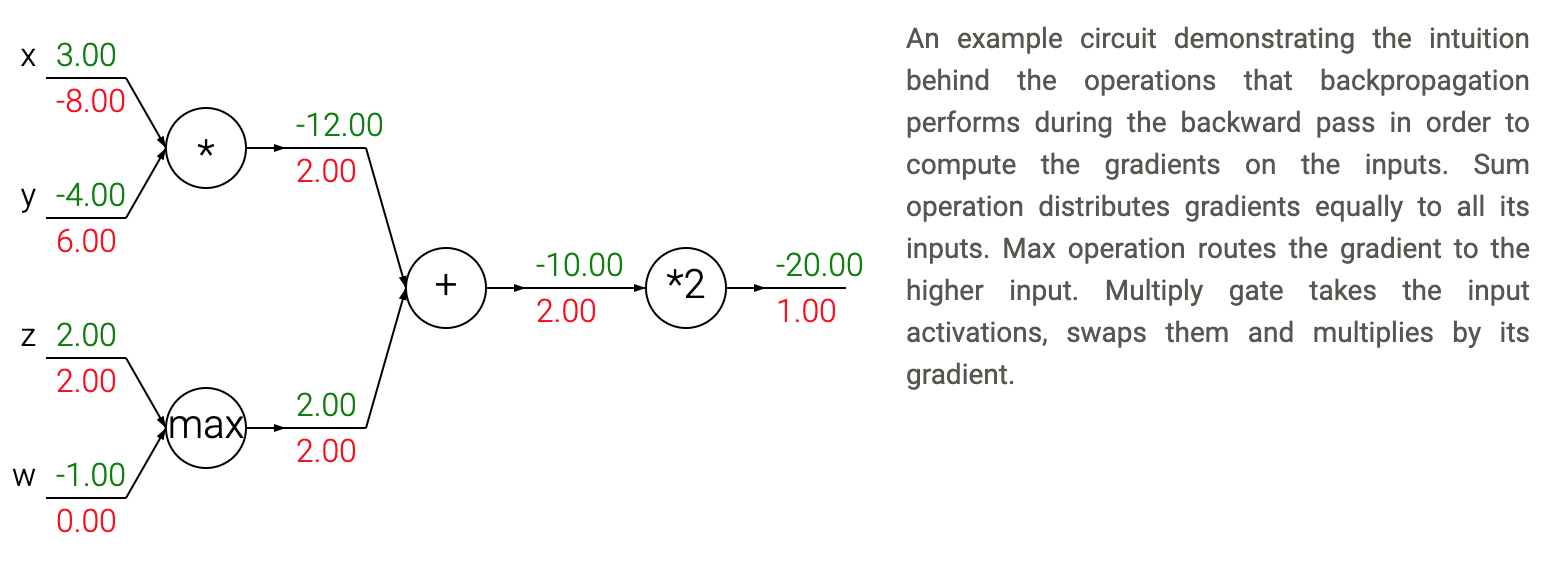The circuit diagram above shows a computation graph which allows to relate the value and gradient of the variables to the functions that produced them. In order to achieve this we will make 2 constructs that are defined in terms of each other:

• a Variable, which is a wrapper around a tensor. It also stores the originating Function of this Variable, if any. For example, the function of Variable x will be None if x = Variable(ns.zeros(3, 3,)), but will be Mul if x = a * b, where a and b are also Variables.
• a Function, which has a forward() method that produces the standard result of a computation, and a backward(g) method where g is a tensor that represents the upstream gradient. The gradient is distributed among the composing Variables according to the rules of calculus, and passed on to the backward(g) methods of these Variables.

### Variables & Functions¶

In :
/**
* Wrapper around a tensor.
* Keeps track of the computation graph by storing the originating function of this variable, if any.
* The function will be None if the variable is the result of simple wrapping around a tensor.
* If the variable is the result of a computation, then f will be set to the function behind this computation.
* For example, f will be None if x = Variable(ns.zeros(3, 3,)), but f will be Mul if x = a * b,
* where a and b are also Variables.
*
* @param data the tensor
* @param f function that produced this variable, if any.
*/
case class Variable(data: Tensor, f: Option[Function] = None) {

/* the local gradient */
lazy val g: Tensor = ns.zerosLike(data)

/**
* Accumulates the incoming gradient in the local gradient.
* Pushes the incoming gradient back through the network,
* by means of the originating function, if any.
* The gradient defaults to 1, as per the Calculus definition.
* @param gradOutput the gradient that is being pushed back through the network
*/
def backward(gradOutput: Tensor = ns.ones(data.shape)): Unit = {
// squash the dimensions to fit the original shape

// If the forward expression involves the variables x,y multiple times,
// then when we perform backpropagation we must be careful to use += instead of =
// to accumulate the gradient on these variables (otherwise we would overwrite it).
// This follows the multivariable chain rule in Calculus,
// which states that if a variable branches out to different parts of the circuit,
// then the gradients that flow back to it will add.
// http://cs231n.github.io/optimization-2/#staged
g += ug

// backprop thru the function that generated this variable, if any
for (gf <- f) gf.backward(ug)
}

def +(other: Variable): Variable = Add(this, other).forward()
def *(other: Variable): Variable = Mul(this, other).forward()
def dot(other: Variable): Variable = Dot(this, other).forward()
def t(): Variable = Transpose(this).forward()

// chain operator, allows to attach variables to functions without using call syntax
def ~>(f: Variable => Variable): Variable = f(this)
def shape: List[Int] = data.shape.toList

def unbroadcast(t: Tensor): Tensor =
if (t.shape.sameElements(data.shape)) t
else data.shape.zip(t.shape).zipWithIndex.foldLeft(t) {
case (d: Tensor, ((oi, ni), i)) if oi == ni =>
d
case (d: Tensor, ((oi, ni), i)) if oi == 1 =>
ns.sum(d, axis = i)
case _ =>
throw new Exception(
s"unable to reduce broadcasted shape ${t.shape.toList} as${data.shape.toList}")
}
}

object Variable {
// turn a sequence of numbers directly into a Variable
def apply(ds: Double*): Variable = Variable(Tensor(ds:_*))
}

/**
* The Function is the 2nd required component in keeping track of a computation graph.
* It defines 2 methods:
*   forward(): produces the traditional result of a computation
*   backward(g): takes a gradient and distributes it to the composing
*     Variables of the Function by calling their backward(g) method.
*/
trait Function {
def forward(): Variable
def backward(g: Tensor): Unit
}

/* Elementwise addition of 2 tensors */
case class Add(v1: Variable, v2: Variable) extends Function {
def forward(): Variable = Variable(v1.data + v2.data, f = Some(this))
/* The Add function distributes its gradient equally to all the inputs */
def backward(g: Tensor): Unit = {
v1.backward(g)
v2.backward(g)
}
}

/* Elementwise multiplication of 2 tensors */
case class Mul(v1: Variable, v2: Variable) extends Function {
override def forward(): Variable = Variable(v1.data * v2.data, f = Some(this))
/* The Mul function swaps the input activations, and multiplies them with the gradient */
override def backward(g: Tensor): Unit = {
val dv2 = v2.data * g
val dv1 = v1.data * g
v1.backward(dv2)
v2.backward(dv1)
}
}

/* Dot product of 2 tensors */
case class Dot(v1: Variable, v2: Variable) extends Function {
val w: Tensor = v1.data
val x: Tensor = v2.data
override def forward(): Variable = Variable(w dot x, f = Some(this))
override def backward(g: Tensor): Unit = {
val dw = g dot x.T
val dx = w.T dot g
v1.backward(dw)
v2.backward(dx)
}
}

/* Transpose a tensor */
case class Transpose(v: Variable) extends Function {
override def forward(): Variable = Variable(v.data.transpose, Some(this))
override def backward(gradOutput: Tensor): Unit =
}


### Activation¶

Activation functions are element-wise functions that introduce a non-linearity in the network. RELU is the most commonly used activation. In the forward pass, it simply sets all negative values in a tensor equal to zero. In the backward pass, it routes the gradient to the input higher than 0.

In :
case class Threshold(x: Variable, d: Double) extends Function {
override def forward(): Variable = Variable(ns.maximum(x.data, d), Some(this))
override def backward(gradOutput: Tensor): Unit = {
x.backward(gradOutput * (x.data > d))
}
}

def relu(x: Variable): Variable = Threshold(x, 0.0).forward()


### Module¶

A module is a wrapper around a set of Variables, here called parameters because they constitute the layers of a network.  When layers are stacked on top of each other, the encompassing module allows to dynamically pull up the parameters of its layers. This can be done by calling the parameters method. In the same way the gradients of all the layers can be pulled up through method gradients. The zeroGrad method allows to set all gradients of the parameters equal to 0. The Module behaves like a Function through the abstract methods forward() and apply().

In :
abstract class Module(localParameters: Seq[Variable] = Nil) {
// by default, obtain submodules through introspection
lazy val subModules: Seq[Module] =
this.getClass.getDeclaredFields.flatMap { f =>
f setAccessible true
f.get(this) match {
case module: Module => Some(module)
case _ => None
}
}

def parameters: Seq[Variable] = localParameters ++ subModules.flatMap(_.parameters)
def gradients: Seq[Tensor] = parameters.map(_.g)
def zeroGrad(): Unit = parameters.foreach(p => p.g := 0)

def forward(x: Variable): Variable
def apply(x: Variable): Variable = forward(x)
}

/* extend module to act like a Function/Variable */
implicit def moduleApply[T <: Module](m: T): (Variable) => Variable = m.forward


#### Linear Module¶

Applies a linear transformation (dot product) to its input.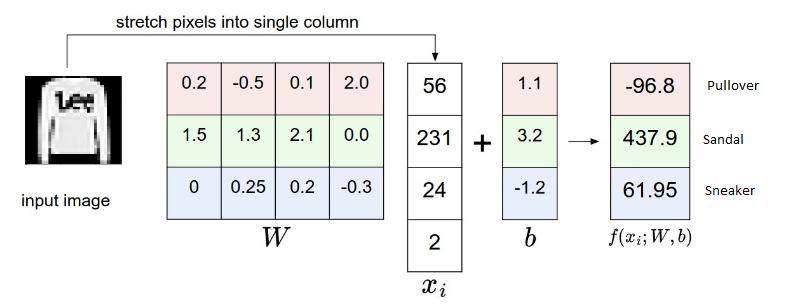In :
case class Linear(weights: Variable, bias: Variable)
extends Module(Seq(weights, bias)) {
override def forward(x: Variable): Variable = {
(x dot weights.t()) + bias
}
}

object Linear {
def apply(inFeatures: Int, outFeatures: Int): Linear = {
/* He initialization */
val w = ns.randn(outFeatures, inFeatures) * math.sqrt(2.0 / (inFeatures + outFeatures))
val weights = Variable(w)
val b = ns.zeros(1, outFeatures)
val bias = Variable(b)
Linear(weights, bias)
}
}


### Optimizer¶

The task of an Optimizer is to nudge the parameters into the direction of the expected outcome of the model. At each step (epoch) it will be called to perform a function on the parameters, using their gradients, in order to achieve this. The Optimizer also provides a method to reset the gradients to 0, which should be called after each update of the parameters.

In :
abstract class Optimizer(parameters: Seq[Variable]) {
def step(epoch: Int)
def zeroGrad(): Unit = parameters.foreach(p => p.g := 0)
}


#### Stochastic Gradient Descent¶

SGD is a type of Optimizer, that implements a simple yet very efficient approach to discriminative learning of a linear classifier. It subtracts a fraction of the gradient of a parameter from the parameter itself. This fraction is called the learning rate.

In :
case class SGD(parameters: Seq[Variable], learningRate: Double) extends Optimizer(parameters) {
override def step(epoch: Int): Unit =
parameters.foreach { p =>
p.data -= learningRate * p.g
}
}


### Softmax Loss function¶

The loss function quantifies our unhappiness with the score of the network. It measures the error between the predicted and the expected value. Intuitively, the loss will be high if we’re doing a poor job of classifying the training data, and it will be low if we’re doing well.

The goal of the network is to find parameters that minimize the loss over all samples.

For a classification task, SoftMax Loss is often used used. It is a combination of a Softmax activation and a Cross Entropy Loss function. Usually they are combined into one function.

Softmax squashes the raw class scores into normalized positive values that sum to one. See also http://neuralnetworksanddeeplearning.com/chap3.html#softmax

In :
case class SoftmaxLoss(actual: Variable, target: Variable) extends Function {
val x: Tensor = actual.data
val y: Tensor = target.data.T

val shiftedLogits: Tensor = x - ns.max(x, axis = 1)
val z: Tensor = ns.sum(ns.exp(shiftedLogits), axis = 1)
val logProbs: Tensor = shiftedLogits - ns.log(z)
val n: Int = x.shape.head
val loss: Double = -ns.sum(logProbs(ns.arange(n), y)) / n

override def forward(): Variable = Variable(Tensor(loss), Some(this))

override def backward(gradOutput: Tensor /* not used */ ): Unit = {
val dx = ns.exp(logProbs)
dx(ns.arange(n), y) -= 1
dx /= n

actual.backward(dx)
}
}

type LossFunction = (Variable, Variable) => Variable

val softmaxLoss: LossFunction = (yHat: Variable, y: Variable) => SoftmaxLoss(yHat, y).forward


In :
trait DataLoader extends Iterable[(Variable, Variable)] {
def numSamples: Int
def numBatches: Int
def mode: String
def iterator: Iterator[(Variable, Variable)]
}


#### Fashion MNIST data loader¶

This dataloader reads the Fashion MNIST dataset from Zalando: https://github.com/zalandoresearch/fashion-mnist. The dataset in CSV format can be downloaded from Kaggle : https://www.kaggle.com/zalando-research/fashionmnist. In the cell below, I have defined a variable baseFolder which points to the download folder. Note that I also assume that the files have been gzipped.

Each line in the file represents an image and its label. There are 10 classes: t-shirt, trousers, pullover, dress, coat, sandal, shirt, sneaker, bag and ankle boot. It is a dataset with 60K training images and 10K validation images.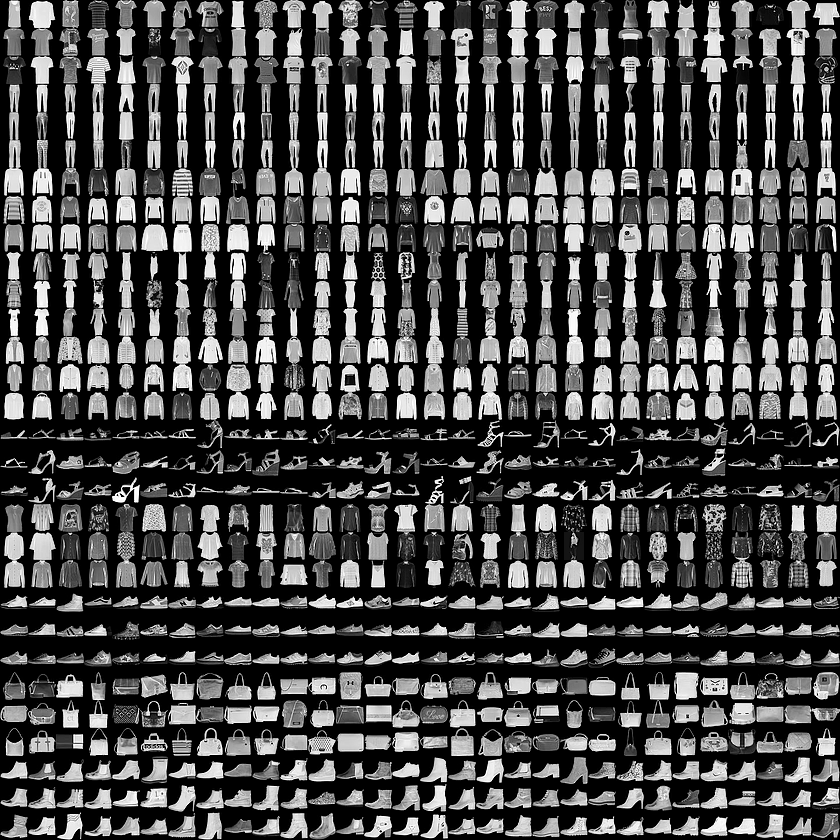Each image consists of 28x28 grayscale pixels. The values of the pixels in the files are all integers between 0 and 255.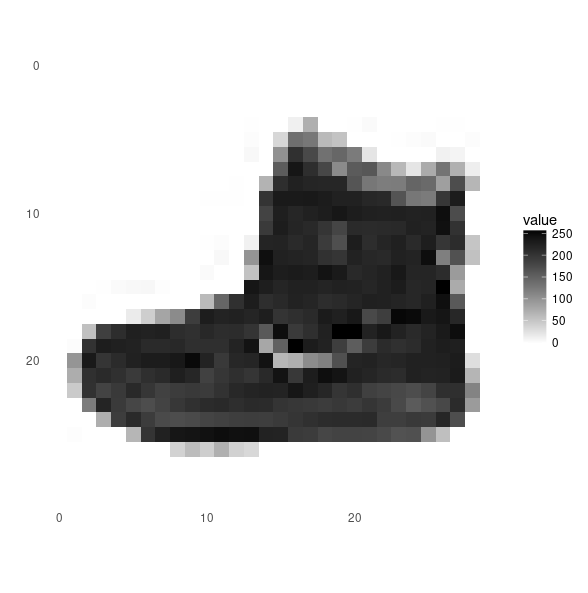The dataloader turns the values into floats between 0 and 1 by dividing the values for the pixels by 255.0. It also applies zero centering: subtract the mean across every individual feature in the data. The geometric interpretation for this is to center the data around the origin along every dimension.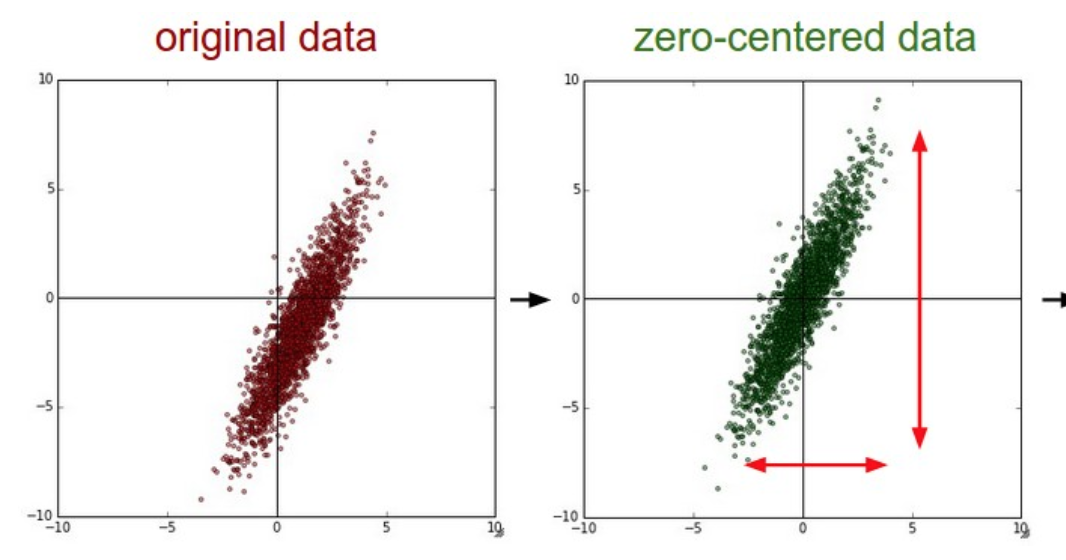Caveat: when zero-centering test data, the mean of the training data must be subtracted from the test data.

In :
import scala.io.Source
import scala.language.postfixOps
import scala.util.Random
import java.util.zip.GZIPInputStream
import java.io._

val baseFolder ="dl-from-scratch-polynb/data/fashionmnist"

class FashionMnistDataLoader(val mode: String,
miniBatchSize: Int,
take: Option[Int] = None,
seed: Long = 231)

Random.setSeed(seed)

val file: String = mode match {
case "train" => s"$baseFolder/fashion-mnist_train.csv.gz" case "valid" => s"$baseFolder/fashion-mnist_test.csv.gz"
}

def gis(f: String) = Source.fromInputStream(
new GZIPInputStream(new BufferedInputStream(new FileInputStream(f))))

val lines: List[String] = {
val src = gis(file)
val lines = src.getLines().toList
src.close()
Random.shuffle(lines.tail) // skip header
}

val numFeatures = 784
val numEntries: Int = lines.length

val numSamples: Int = take match {
case Some(n) => math.min(n, numEntries)
case None    => numEntries
}

val numBatches: Int =
(numSamples / miniBatchSize) +
(if (numSamples % miniBatchSize == 0) 0 else 1)

val data: Seq[(Variable, Variable)] = lines
.take(take.getOrElse(numSamples))
.sliding(miniBatchSize, miniBatchSize)
.map { lines =>
val batchSize = lines.length

val xs = Array.fill[Float](numFeatures * batchSize)(elem = 0)
val ys = Array.fill[Float](batchSize)(elem = 0)

lines.zipWithIndex.foreach {
case (line, lnr) =>
val tokens = line.split(",")
tokens.tail.zipWithIndex.foreach {
case (sx, i) =>
xs(lnr * numFeatures + i) = sx.toFloat / 255.0f
}
}

val x = Variable(Tensor(xs).reshape(batchSize, numFeatures))
val y = Variable(Tensor(ys).reshape(batchSize, 1))
(x, y)
}
.toSeq

lazy val meanImage: Tensor = {
val m = ns.zeros(1, numFeatures)
data.foreach {
case (x, _) =>
m += ns.sum(x.data, axis = 0)
}
m /= numSamples
m
}

if (mode == "train") zeroCenter(meanImage)

def zeroCenter(meanImage: Tensor): Unit = {
val bcm = broadcastTo(meanImage, miniBatchSize, numFeatures)

data.foreach {
case (x, _) =>
if (x.data.shape.head == miniBatchSize)
x.data -= bcm
else
x.data -= meanImage
}
}

def iterator: Iterator[(Variable, Variable)] =
Random.shuffle(data.toIterator)

def broadcastTo(t: Tensor, shape: Int*): Tensor =

}


### Evaluator¶

After each epoch we want to evaluate the model, so that we can follow how good or how bad it is doing.

The metrics we're computing in the evaluator below are the loss (which we want to be low) and the accuracy (which we want to be high). We will collect the metrics of all minibatches of the training and validation data set. This will allow us to visualize the performance of the model.

In :
trait Evaluator {
def evaluate(epoch: Int, startTime: Long, endTime: Long, net: Module, loss: LossFunction): Unit
}

loss: LossFunction) extends Evaluator {
import scala.collection.mutable.ArrayBuffer
val trnLossMetrics = ArrayBuffer[Float]()
val valLossMetrics = ArrayBuffer[Float]()
val trnAccuracyMetrics = ArrayBuffer[Float]()
val valAccuracyMetrics = ArrayBuffer[Float]()

def metrics = (
trnLossMetrics.toArray,
valLossMetrics.toArray,
trnAccuracyMetrics.toArray,
valAccuracyMetrics.toArray)

def evaluate(epoch: Int, t0: Long, t1: Long, net: Module, loss: LossFunction): Unit = {
val dur = (t1 - t0) / 1000000
val (ltrn, atrn) = evaluate(trainDataLoader, net, trnLossMetrics, trnAccuracyMetrics)
val (lval, aval) = evaluate(validDataLoader, net, valLossMetrics, valAccuracyMetrics)
println(
f"epoch: $epoch%2d duration:$dur%4dms loss: $lval%1.4f /$ltrn%1.4f\taccuracy: $aval%1.4f /$atrn%1.4f")
}

net: Module,
lossMetrics: ArrayBuffer[Float],
accuracyMetrics: ArrayBuffer[Float]): (Double, Double) = {
val (l, a) =
dl.foldLeft(0.0, 0.0) {
case ((lossAcc, accuracyAcc), (x, y)) =>
val output = net(x)
val guessed = ns.argmax(output.data, axis = 1)
val accuracy = ns.sum(guessed == y.data) / x.shape.head
val cost = loss(output, y).data.squeeze()
lossMetrics += cost.toFloat
accuracyMetrics += accuracy.toFloat
(lossAcc + cost, accuracyAcc + accuracy)
}
(l / dl.numBatches, a / dl.numBatches)
}
}


### Learner¶

Convenience class around the learning process. You provide it with the training datasets, the model layout to train, an optimizer, a loss function, and an evaluator.

The class provides a fit method, that will train the model for the required number of epochs.

For  a given epoch, the fit method will loop through all the minibatches of the training set, and for each minibatch will perform the following operations:

• set the gradients of all parameters of the network to 0
• perform a forward pass on the input
• perform the loss function on the outcome of the forward pass
• perform the backward pass on the outcome of the loss function
• perform an optimization step

The model is evaluated after each epoch.

In :
case class Learner(trainingDataLoader: DataLoader,
net: Module,
optimizer: Optimizer,
loss: LossFunction,
evaluator: Evaluator) {
var epochs = 0
def fit(numEpochs: Int): Unit = {
(0 until numEpochs) foreach { _ =>
val t0 = System.nanoTime()
trainingDataLoader.foreach { // for each mini batch
case (x, y) =>
val yh = net(x)
val l = loss(yh, y)
l.backward()
optimizer.step(epochs) // update parameters using their gradient
}
val t1 = System.nanoTime()
evaluator.evaluate(epochs, t0, t1, net, loss)
epochs += 1
}
}
}


### Train a network¶

Create the data loaders for the training and validation data sets. Zero-center the validation set with the mean image of the training set.

The instantiation of the dataloaders may take some time.

In :
val batchSize = 256
val trainDl = new FashionMnistDataLoader("train", batchSize)
val validDl = new FashionMnistDataLoader("valid", batchSize)
validDl.zeroCenter(trainDl.meanImage)


In the next cell we define the layout of our network: 2 linear transformations with a RELU activation in between.

In :
val nn: Module = new Module() {
val fc1 = Linear(784, 100)
val fc2 = Linear(100, 10)
override def forward(x: Variable): Variable =
x ~> fc1 ~> relu ~> fc2
}


Next, we define the optimizer for the given learning rate, and the number of epochs we want to train the model. Then start the training.

You should not expect state-of-the-art results with this simple network. It just proves that the model can learn.

In :
val learningRate = .1
val sgd = SGD(nn.parameters, learningRate)
val eval = MetricsCollector(trainDl, validDl, softmaxLoss)
val learner = Learner(trainDl, nn, sgd, softmaxLoss, eval)

In :
val numEpochs = 10
learner.fit(numEpochs)

epoch:  0 duration: 5052ms loss: 0.5210 / 0.5223	accuracy: 0.8193 / 0.8170
epoch:  1 duration: 4401ms loss: 0.4506 / 0.4510	accuracy: 0.8443 / 0.8413
epoch:  2 duration: 4308ms loss: 0.4212 / 0.4174	accuracy: 0.8530 / 0.8526
epoch:  3 duration: 4302ms loss: 0.4033 / 0.3985	accuracy: 0.8590 / 0.8594
epoch:  4 duration: 4239ms loss: 0.3876 / 0.3803	accuracy: 0.8642 / 0.8661
epoch:  5 duration: 4267ms loss: 0.3748 / 0.3663	accuracy: 0.8665 / 0.8699
epoch:  6 duration: 4251ms loss: 0.3682 / 0.3557	accuracy: 0.8669 / 0.8740
epoch:  7 duration: 4252ms loss: 0.3592 / 0.3458	accuracy: 0.8706 / 0.8778
epoch:  8 duration: 4273ms loss: 0.3546 / 0.3392	accuracy: 0.8714 / 0.8787
epoch:  9 duration: 4375ms loss: 0.3514 / 0.3310	accuracy: 0.8740 / 0.8832


### Visualize loss and accuracy¶

In the next cells we will make use of a unique feature of polynote, which, as its name suggests, provides the possibility to mix languages in the same notebook, and what is really interesting, to use variables created in one cell in another cell of a different language. We will prepare the Scala variables in the cell below, and then use them for visualization by matplotlib in the following Python cell.

In :
val (tl, vl, ta, va) = eval.metrics

In :
import matplotlib.pyplot as plt
import numpy as np

plt.close()

def plot_metrics(metrics, label):
fig, ax = plt.subplots()
ax.plot(metrics, 'b')
ax.set(xlabel='minibatch', ylabel=label, title=label)
ax.grid()
plt.plot(np.arange(len(metrics)), metrics)

plot_metrics(tl, 'training loss')
plot_metrics(vl, 'validation loss')
plot_metrics(ta, 'training accuracy')
plot_metrics(va, 'validation accuracy')

plt.show()

In :